x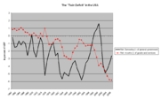Twin deficit hypothesisEncyclopedia
The twin deficits hypothesis, also called the double deficit hypothesis or twin deficits anomaly, is a concept from macroeconomics
Macroeconomics
Macroeconomics is a branch of economics dealing with the performance, structure, behavior, and decision-making of the whole economy. This includes a national, regional, or global economy...

that contends that there is a strong link between a national economy's current account balance and its government budget balance.

## Definition

Macroeconomic theory points to a link between the budget balance and the current account balance.

This link can be seen from considering the National accounting model of the economy: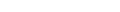where Y represents National Income or GDP, C is consumption, I is investment, G is government spending and NX stands for net exports. This represents GDP because all the production in an economy (the left hand side of the equation) is used as consumption (C), investment (I), or government spending (G), and the leftover production is exported (NX). Another equation defining GDP using alternative terms (which in theory results in the same value) is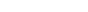where Y is again GDP, C is consumption, S is savings, and T is taxes. This is because national income is also equal to output, and all individual income either goes to pay for consumption (C), to pay taxes (T), or becomes savings (S).

## Proof

Since, and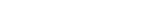, then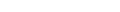, which simplifies to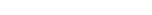If (T-G) is negative, we have a budget deficit.

Now, assume an economy already at potential output, meaning Y is fixed. In this case, if the deficit increases, and savings remains the same, then this last equation implies that either investment (I) must fall (see crowding out
Crowding out (economics)
In economics, crowding out occurs when Expansionary Fiscal Policy causes interest rates to rise, thereby reducing private spending. That means increase in government spending crowds out investment spending....

), or net exports (NX) must fall, causing a trade deficit. Hence, the twin deficits.

Though the economics behind which of the two is used to finance the government deficit can get more complicated than what is shown above, the essence of it is that if foreigners' savings pay for the budget deficit, the current account deficit grows. If the countries own citizens' savings finance the borrowing, it may cause a crowding out effect (in an economy at or near potential output, or full employment).

## Example

In the US, the budget deficit is financed about half from foreigners, half domestically. Therefore, with a \$400 billion deficit, the trade deficit would, according to the theory, be increased by some \$200 billion.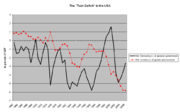An economy
Economic system
An economic system is the combination of the various agencies, entities that provide the economic structure that defines the social community. These agencies are joined by lines of trade and exchange along which goods, money etc. are continuously flowing. An example of such a system for a closed...

is deemed to have a double deficit if it has a current account
Current account
In economics, the current account is one of the two primary components of the balance of payments, the other being the capital account. The current account is the sum of the balance of trade , net factor income and net transfer payments .The current account balance is one of two major...

deficit and a fiscal deficit. In effect, the economy is borrowing from foreigners in exchange for foreign-made goods. Traditional macroeconomics
Macroeconomics
Macroeconomics is a branch of economics dealing with the performance, structure, behavior, and decision-making of the whole economy. This includes a national, regional, or global economy...

predicts that persistent double deficits will lead to currency devaluation
Devaluation
Devaluation is a reduction in the value of a currency with respect to those goods, services or other monetary units with which that currency can be exchanged....

/depreciation
Depreciation
Depreciation refers to two very different but related concepts:# the decrease in value of assets , and# the allocation of the cost of assets to periods in which the assets are used ....

that can be severe and sudden.

In the case of the United States, the twin deficit graph as a percentage of GDP shows that the budget and current account deficits did move broadly in sync from 1981 until the early 1990s, but since then, they have moved apart. Data thus confirm that as a government budget deficit widens, the current account falls, but the relationship is complicated by what happens to investment and private saving.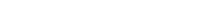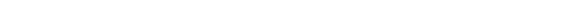Above the equation, it's verifiable that CA will deteriorate as government expenditure exceeds the amount of tax is collected.

One way to understand this process intuitively is by thinking through two different markets. First consider the Foreign Exchange market. At equilibrium the quantity supplied=the quantity demanded. Thus, Imports + Capital outflow=Exports + Capital Inflow. Rearranging this equation we find that Imports-Exports=Capital Inflow-Capital Outflow. Because Imports-Exports=Trade Deficit and Capital Inflow-Capital Outflow=Net Capital Inflow, we get the equation Trade Deficit=Net Capital Inflow (or Current Account deficit = Capital Account Surplus).

Next we must consider the market for loanable funds. The equilibrium here is Savings+Net Capital Inflow=Investment+Budget Deficit. However, taking the Forex market into consideration we know that the Trade Deficit is equal to Net Capital Inflow. We can thus substitute for: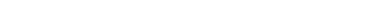Rearranging algebraically we find that: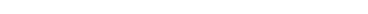What we can gather from this is the understanding of why an increased budget deficit goes up and down in tandem with the Trade Deficit. This is where we derive the appellation the Twin Deficits: if the US budget deficit goes up then either household savings must go up, the trade deficit must go up, or private investment will decrease.
The source of this article is wikipedia, the free encyclopedia.  The text of this article is licensed under the GFDL.